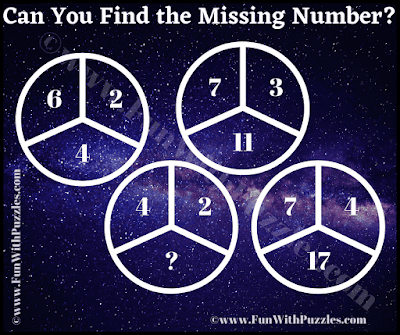It is a tricky maths puzzle for high school students. Solving this maths puzzle will test your mathematical skills and logical skills. In this circle math puzzle, you are shown 3 numbers inside the Circle. These numbers relate to each other mathematical. Your challenge to crack the hidden code which relate these given numbers in the Circle. Once you are able to crack the code, then find the value of the missing number in the Circle.Can you find the value of the missing number?

The answer to this "Tricky Maths Puzzle", can be viewed by clicking on the answer button.